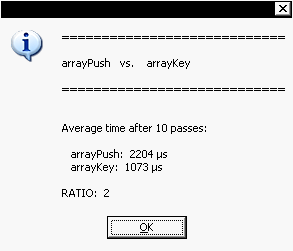Is it faster to use `myArray.push(x)` or `myArray[myArray.length]=x`? What is the effectiveness of `~~x` over `Math.round(x)`? Should you prefer `String` concatenation or `Array.join('')`? Is it better to directly traverse a DOM `Collection` or to loop into the true array:
`myCollection.everyItem().getElements()`?

There are many circumstances where very small local enhancements can dramatically speed up the global execution time of an InDesign script. Each time you implement a routine that will be repeatedly —or recursively!— invoked, there is much to gain in testing its performance.

Thanks to `\$.hiresTimer` it is very easy to time a code snippet. According to the documentation `\$.hiresTimer` is a “high-resolution timer, measuring the time in microseconds. The timer starts when ExtendScript is initialized during the application startup sequence. Every read access resets the timer to zero.”

## ComparePerf.js

ComparePerf.js is a simple script that allows you to compare time performance of two competing functions. It is based on the following piece:

```function perf(/*int*/n, /*func*/f /*,arg1,arg2,...*/)
//--------------------------------------
{
var args =  Array.prototype.slice.call(arguments, 2);

var i = (n=n||1), t, r = 0;

while(i--)
{
\$.hiresTimer;
f.apply(null, args);
t = \$.hiresTimer;
r += t;
}

// Clean up
// ---
args.length = 0;
args = null;

// Average time
// ---
return ~~(r/n);
};
```

Then we just need to write an interface —`comparePerf()`— that supplies each function to `perf()` and formats the measured times:

```function comparePerf(/*func*/f1, /*func*/f2 /*,arg1,arg2,...*/)
//--------------------------------------
{
var args =  Array.prototype.slice.call(arguments, 2),
n = comparePerf.PASSES;

var t1 = perf.apply(null, [n, f1].concat(args)),
t2 = perf.apply(null, [n, f2].concat(args)),
r = .1 * ~~(10*(t1/t2));

// Clean up
// ---
args.length = 0;
args = null;

f1.name + '   vs.    ' + f2.name + '\r\r' +
'============================\r\r\r' +
'Average time after ' + n + ' passes:\r\r' +
'   ' + f1.name + ':  ' + t1 + ' \xB5s\r' +
'   ' + f2.name + ':  ' + t2 + ' \xB5s\r\r' +
'RATIO:  ' + r );

};

comparePerf.PASSES = 10;
```

Above is the heart of the script. Now, let's compare two sample functions:

```// . . .
// SAMPLE TEST

function arrayPush()
{
var a = [], n = 1000;
while( n-- ) a.push(1);
};

function arrayKey()
{
var a = [], n = 1000;

var i = -1;
while( n-- ) a[++i] = 1;
};

comparePerf(arrayPush, arrayKey);
```

As my computer is prehistoric you surely will get better absolute times, but the ratio should be similar to what is displayed below:Well. It appears that our `arrayKey()` function is 2X faster than `ArrayPush()`!

Notes. — If funcA and funcB require additional arguments, you can use the syntax: `comparePerf(funcA, funcB, arg1, arg2...)`. The script then compares `funcA(arg1, arg2)` and `funcB(arg1, arg2)`. Furthermore, you can change the value of `comparePerf.PASSES` —default: 10— in order to increase test iterations. This might significantly refine your benchmark.

Enjoy!